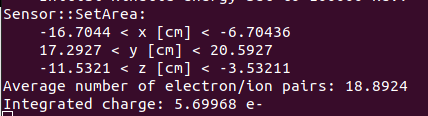# The questions about the integrated charge

as in the screen shot, why the average electron/ion pairs are 18.8924 per cm, the thickness of my detector is 10mm, then the integrated charge(the number of electrons) would be 18.8924, then why the integrated charge is 5.69968?Does that mean when one proton traverse into the detector, it would generate 5.69968 electrons in average?

1 Like

Hi,
as discussed before, the integrated signal does converge to the deposited charge, but only if you integrate over a long enough time to catch both the electron and the ion signal.

1 Like

Ok,I understand, That means if the time is long enough , the number of the electron would be 18.8924.

Yes, exactly.

1 Like

This topic was automatically closed 14 days after the last reply. New replies are no longer allowed.